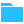#Context Library (PQL)

• Family: PQL
• Context functions extend the original semantic calculation model to include the reporting context in the method's resolution.

By including the context in the 'equation', these calculation types can better adapt to changing report designs, query elements, and structures WITHOUT any need to rewrite the formulations for each variation. For example, calculations can include 'context' logic that compares each row to the previous row in a query result to find the rolling difference values. While one-off calculations in the semantic layer can achieve the same outcome, they cannot adapt to changes in the query easily.

Context calculations are usually done in TOOLS like Excel rather than in languages.

As described in the PQL Calculation Overview, context functions are part of a broad set of methods and capabilities for infusing your analytics with mathematical operations and logic. They can be used in conjunction with the Common function library to build deep, sophisticated methods to resolve analytical problems.

### Writing or Editing Context Methods

Context functions can only be created and driven through the context menus in Discover. They cannot be edited or created from scratch in Formulate.

## Function Listing

##### Triggered from the context menus in Discover

PQL Function

Syntax

Description

Average

Avg

Calculates the average of the supplied value / measure across the current result set.

Bin by Percentile

PercentileBin

Generates a new attribute. First, it orders all data points for the supplied value / measure across the current result set. It then bins the cells into percentile ranges to produce the generated binning attribute.

Bin by Rank

RankBin

Generates a new attribute. First, it orders all data points for the supplied value / measure across the current result set. It then bins the cells into ranges based on a high-to-low ranked ordering to produce the generated binning attribute.

Cumulative Totals

Cumulative

Calculates the accumulating total of the supplied value / measure across the current result set.

Difference

Diff

Calculates the differential of the supplied value / measure across the current result set cell by cell. Choosing the "direction" of the calculation allows users to determine the cell-by-cell ordering.

Exponential Regression

ExpReg

Calculates the linear regression (using an exponential equation) for the supplied value / measure across the current result set. If there are no other values, the regression is designed against the ordinal of the cells. If there are one or more other values, the regression is calculated using those other measures in a variate or multivariate formulation.

Linear Regression

LinReg

Calculates the linear regression (using a linear equation) for the supplied value / measure across the current result set. If there are no other values, the regression is designed against the ordinal of the cells. If there are one or more other values, the regression is calculated using those other measures in a variate or multivariate formulation.

Linear regression Outliers

linregoutliers

Related to the linear regression calculation, except items that are 2 or more standard deviations from the regressional mean are designated "out" versus "in".

Logarithmic Regression

LogReg

Calculates the linear regression (using a logarithmic equation) for the supplied value / measure across the current result set. If there are no other values, the regression is designed against the ordinal of the cells. If there are one or more other values, the regression is calculated using those other measures in a variate or multivariate formulation.

Pareto

Pareto

Calculates the accumulating total as a percentage of the grand total for the supplied value / measure across the current result set.

Percent Difference

PercentDiff

Calculates the percent differential of the supplied value / measure across the current result set cell by cell. Choosing the "direction" of the calculation allows users to determine the cell-by-cell ordering.

Percent of

Percent

Calculates the percent change of the supplied value / measure across the current result set cell by cell. Choosing the "direction" of the calculation allows users to determine the cell-by-cell ordering.

Percent of Max

PercentofMax

Calculates the percent of a supplied value / measure cell in the context of the maximum value.

Percent of Total

PercentofTotal

Calculates the percent of a supplied value / measure cell in the context of the total value.

Power Regression

PowReg

Calculates the linear regression (using a power equation) for the supplied value / measure across the current result set. If there are no other values, the regression is designed against the ordinal of the cells. If there are one or more other values, the regression is calculated using those other measures in a variate or multivariate formulation.

Standard Deviation

StdDev

Calculates the specified standard deviation of the supplied value / measure across the current result set.

Outliers

StdDevOutliers

Generates a new attribute. First, it calculates the specified standard deviation of the supplied value / measure across the current result set. Any elements above / below 2 standard deviations are flagged as an outlier, versus an in-lier in the generated outlier attribute.

##### Triggered from the alternative locations in Discover

PQL Function

Syntax

Description

Geo Boundary

GeoBound

Triggered from a geospatial map visual by the lasso tool, this will find all data points within the specified distance range of the center and group them into 2 lists of "in" and "out" members.

Outliers from Ribbon

MultiVarOutliers

Triggered from the query tab, it generates a new attribute. First, it calculates the specified standard deviation of the supplied value / measure across the current result set. If there are 2 measures, the standard deviation is measured against the regressed mean of the 2 measures. If there are 3 or more measures, the standard deviation is measured against the multivariate regressional mean.